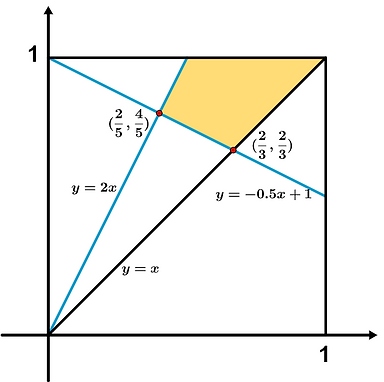Solution of the Week:

3 Vertices, 2 Midpoints

The blue segments connect a vertex to the midpoint of a side. What fraction of the square's area is represented by the yellow quadrilateral?

Solution:

1. The yellow area together with the triangles on its right and left represent a quarter of the square's area.

2. Find the equation for the 3 segments. Their intersection points help us find the heights of the two triangles.